# Rotary cylinder

The rotating cylinder has a surface area of 69.08 cm2. The area of the shell is 62.8 cm 2. What is the diameter of the cylinder?

D =  2 cm

### Step-by-step explanation: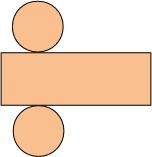We will be pleased if You send us any improvements to this math problem. Thank you!#### You need to know the following knowledge to solve this word math problem:

We encourage you to watch this tutorial video on this math problem:

## Related math problems and questions:

• Roller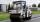Roller has a diameter of 0.96 m and a width 169 cm. How many m2 of road level when he turns 42-times?
• Equilateral cylinderEquilateral cylinder (height = base diameter; h = 2r) has a volume of V = 199 cm3 . Calculate the surface area of the cylinder.
• Surface of the cylinderCalculate the surface of the cylinder for which the shell area is Spl = 20 cm2 and the height v = 3.5 cm
• Tin with oilTin with oil has the shape of a rotating cylinder whose height is equal to the diameter of its base. Canned surface is 1884 cm2. Calculate how many liters of oil is in the tin.
• Height as diameter of baseThe rotary cylinder has a height equal to the base diameter and a surface of 471 cm2. Calculate the volume of a cylinder.
• Rotary bodiesThe rotating cone and the rotary cylinder have the same volume 180 cm3 and the same height v = 15 cm. Which of these two bodies has a larger surface area?
• Axial sectionAxial section of the cylinder has a diagonal 40 cm. The size of the shell and the base surface are in the ratio 3:2. Calculate the volume and surface area of this cylinder.
• Shell area cyThe cylinder has a shell content of 300 cm square, while the height of the cylinder is 12 cm. Calculate the volume of this cylinder.
• The hollow cylinderThe hollow cylinder has a height of 70 cm, an outer diameter of 180 cm, and an inner diameter of 120 cm. What is the surface of the body, including the area inside the cavity?
• Cylinder diameter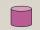The surface of the cylinder is 149 cm2. The cylinder height is 6 cm. What is the diameter of this cylinder?
• Surface area of cylinder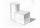Determine the lateral surface of the rotary cylinder which is circumscribed cube with edge length 5 cm.
• Cone containerRotary cone-shaped container has a volume 1000 cubic cm and a height 12 cm. Calculate how much metal we need for making this package.
• What is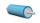What is the height of a cylinder whose surface size is 602.88 cm2 and the content of its shell is 376.8 cm2?
• Lamp coneCalculate the surface of a lamp shade shaped of a rotary truncated cone with base diameter 32 cm and 12 cm and height 24 cm.
• Fit ballWhat is the size of the surface of Gymball (FIT - ball) with a diameter of 65 cm?
• Rotating cone IICalculate area of surface of rotating cone with base radius r=19 cm and height h=9 cm.
• The pipe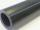The pipe is 1.5 m long. Its outer diameter is 60 cm, inner diameter is 52 cm. Calculate the pipe's weight if the material's density from which it is made is 2 g/cm3. Round the results to whole kilograms.